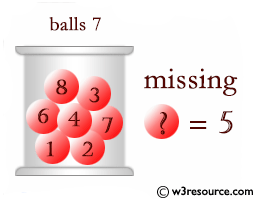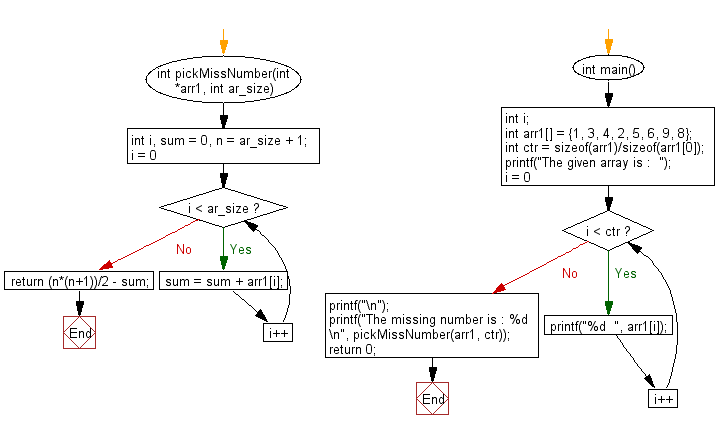﻿ C Program: Find the missing number from a given array - w3resource

# C Exercises: Find the missing number from a given array

## C Array: Exercise-36 with Solution

Write a program in C to find the missing number in a given array. There are no duplicates in the list.

Visual Presentation:Sample Solution:

C Code:

``````#include <stdio.h>

// Function to find the missing number in an array
int pickMissNumber(int *arr1, int ar_size) {
int i, sum = 0, n = ar_size + 1;

// Calculating the sum of all elements in the array
for (i = 0; i < ar_size; i++) {
sum = sum + arr1[i];
}

// Calculating the missing number using the sum of the first 'n' natural numbers formula
return (n * (n + 1)) / 2 - sum;
}

// Main function
int main() {
int i;
int arr1[] = {1, 3, 4, 2, 5, 6, 9, 8};
int ctr = sizeof(arr1) / sizeof(arr1);

// Displaying the given array
printf("The given array is :  ");
for (i = 0; i < ctr; i++) {
printf("%d  ", arr1[i]);
}
printf("\n");

// Finding and displaying the missing number
printf("The missing number is : %d \n", pickMissNumber(arr1, ctr));

return 0;
}
```
```

Sample Output:

```The given array is :  1  3  4  2  5  6  9  8
The missing number is : 7
```

Flowchart :C Programming Code Editor:

What is the difficulty level of this exercise?

Test your Programming skills with w3resource's quiz.

﻿